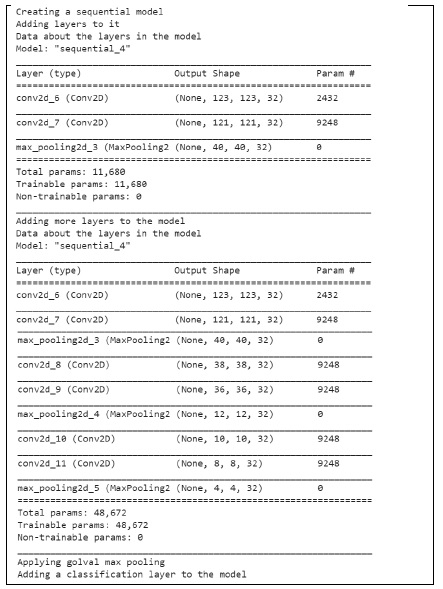# What is a common debugging workflow while creating a model using Keras in Python?

Tensorflow is a machine learning framework that is provided by Google. It is an open-source framework used in conjunction with Python to implement algorithms, deep learning applications and much more. It is used in research and for production purposes. It has optimization techniques that help in performing complicated mathematical operations quickly.

This is because it uses NumPy and multi-dimensional arrays. These multi-dimensional arrays are also known as ‘tensors’.

The ‘TensorFlow’ package can be installed on Windows using the below line of code −

pip install tensorflow

Keras means ‘horn’ in Greek. Keras was developed as a part of the research for the project ONEIROS (Open ended Neuro-Electronic Intelligent Robot Operating System). It runs on top of the Tensorflow framework. It was built to help experiment in a quick manner. It provides essential abstractions and building blocks that are essential in developing and encapsulating machine learning solutions.

It is highly scalable, and comes with cross platform abilities. This means Keras can be run on TPU or clusters of GPUs. Keras models can also be exported to run in a web browser or a mobile phone as well.

Keras is already present within the Tensorflow package. It can be accessed using the below line of code.

import tensorflow
from tensorflow import keras

We are using Google Colaboratory to run the below code. Google Colab or Colaboratory helps run Python code over the browser and requires zero configuration and free access to GPUs (Graphical Processing Units). Colaboratory has been built on top of Jupyter Notebook. Following is the code snippet −

## Example

print("Creating a sequential model")
model = keras.Sequential()
model.add(keras.Input(shape=(250, 250, 3))) # 250x250 RGB images
print("Data about the layers in the model")
model.summary()
print("Adding more layers to the model")
print("Data about the layers in the model")
model.summary()
print("Applying golval max pooling")
print("Adding a classification layer to the model")
model.add(layers.Dense(10))

## Output## Explanation

• When a sequential architecture is being built, it is suggested to incrementally stack layers.

• This can be done using the ‘add’ function.